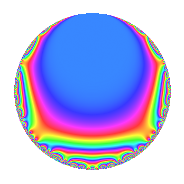Properties

 Label 3311.1.h.iLevel 3311 Weight 1 Character orbit 3311.h Self dual Yes Analytic conductor 1.652 Analytic rank 0 Dimension 2 Projective image $$D_{6}$$ CM disc. -3311 Inner twists 4

Related objects

Newspace parameters

 Level: $$N$$ = $$3311 = 7 \cdot 11 \cdot 43$$ Weight: $$k$$ = $$1$$ Character orbit: $$[\chi]$$ = 3311.h (of order $$2$$ and degree $$1$$)

Newform invariants

 Self dual: Yes Analytic conductor: $$1.65240425683$$ Analytic rank: $$0$$ Dimension: $$2$$ Coefficient field: $$\Q(\sqrt{3})$$ Coefficient ring: $$\Z[a_1, a_2, a_3]$$ Coefficient ring index: $$1$$ Projective image $$D_{6}$$ Projective field Galois closure of 6.0.76739047.1

$q$-expansion

Coefficients of the $$q$$-expansion are expressed in terms of $$\beta = \sqrt{3}$$. We also show the integral $$q$$-expansion of the trace form.

 $$f(q)$$ $$=$$ $$q$$ $$+ q^{2}$$ $$-\beta q^{3}$$ $$-\beta q^{5}$$ $$-\beta q^{6}$$ $$- q^{7}$$ $$- q^{8}$$ $$+ 2 q^{9}$$ $$+O(q^{10})$$ $$q$$ $$+ q^{2}$$ $$-\beta q^{3}$$ $$-\beta q^{5}$$ $$-\beta q^{6}$$ $$- q^{7}$$ $$- q^{8}$$ $$+ 2 q^{9}$$ $$-\beta q^{10}$$ $$- q^{11}$$ $$- q^{14}$$ $$+ 3 q^{15}$$ $$- q^{16}$$ $$-\beta q^{17}$$ $$+ 2 q^{18}$$ $$+ \beta q^{21}$$ $$- q^{22}$$ $$-2 q^{23}$$ $$+ \beta q^{24}$$ $$+ 2 q^{25}$$ $$-\beta q^{27}$$ $$- q^{29}$$ $$+ 3 q^{30}$$ $$+ \beta q^{33}$$ $$-\beta q^{34}$$ $$+ \beta q^{35}$$ $$+ \beta q^{40}$$ $$+ \beta q^{41}$$ $$+ \beta q^{42}$$ $$+ q^{43}$$ $$-2 \beta q^{45}$$ $$-2 q^{46}$$ $$+ \beta q^{48}$$ $$+ q^{49}$$ $$+ 2 q^{50}$$ $$+ 3 q^{51}$$ $$- q^{53}$$ $$-\beta q^{54}$$ $$+ \beta q^{55}$$ $$+ q^{56}$$ $$- q^{58}$$ $$-2 q^{63}$$ $$+ q^{64}$$ $$+ \beta q^{66}$$ $$- q^{67}$$ $$+ 2 \beta q^{69}$$ $$+ \beta q^{70}$$ $$-2 q^{72}$$ $$-2 \beta q^{75}$$ $$+ q^{77}$$ $$+ \beta q^{80}$$ $$+ q^{81}$$ $$+ \beta q^{82}$$ $$-\beta q^{83}$$ $$+ 3 q^{85}$$ $$+ q^{86}$$ $$+ \beta q^{87}$$ $$+ q^{88}$$ $$-2 \beta q^{90}$$ $$+ q^{98}$$ $$-2 q^{99}$$ $$+O(q^{100})$$ $$\operatorname{Tr}(f)(q)$$ $$=$$ $$2q$$ $$\mathstrut +\mathstrut 2q^{2}$$ $$\mathstrut -\mathstrut 2q^{7}$$ $$\mathstrut -\mathstrut 2q^{8}$$ $$\mathstrut +\mathstrut 4q^{9}$$ $$\mathstrut +\mathstrut O(q^{10})$$ $$2q$$ $$\mathstrut +\mathstrut 2q^{2}$$ $$\mathstrut -\mathstrut 2q^{7}$$ $$\mathstrut -\mathstrut 2q^{8}$$ $$\mathstrut +\mathstrut 4q^{9}$$ $$\mathstrut -\mathstrut 2q^{11}$$ $$\mathstrut -\mathstrut 2q^{14}$$ $$\mathstrut +\mathstrut 6q^{15}$$ $$\mathstrut -\mathstrut 2q^{16}$$ $$\mathstrut +\mathstrut 4q^{18}$$ $$\mathstrut -\mathstrut 2q^{22}$$ $$\mathstrut -\mathstrut 4q^{23}$$ $$\mathstrut +\mathstrut 4q^{25}$$ $$\mathstrut -\mathstrut 2q^{29}$$ $$\mathstrut +\mathstrut 6q^{30}$$ $$\mathstrut +\mathstrut 2q^{43}$$ $$\mathstrut -\mathstrut 4q^{46}$$ $$\mathstrut +\mathstrut 2q^{49}$$ $$\mathstrut +\mathstrut 4q^{50}$$ $$\mathstrut +\mathstrut 6q^{51}$$ $$\mathstrut -\mathstrut 2q^{53}$$ $$\mathstrut +\mathstrut 2q^{56}$$ $$\mathstrut -\mathstrut 2q^{58}$$ $$\mathstrut -\mathstrut 4q^{63}$$ $$\mathstrut +\mathstrut 2q^{64}$$ $$\mathstrut -\mathstrut 2q^{67}$$ $$\mathstrut -\mathstrut 4q^{72}$$ $$\mathstrut +\mathstrut 2q^{77}$$ $$\mathstrut +\mathstrut 2q^{81}$$ $$\mathstrut +\mathstrut 6q^{85}$$ $$\mathstrut +\mathstrut 2q^{86}$$ $$\mathstrut +\mathstrut 2q^{88}$$ $$\mathstrut +\mathstrut 2q^{98}$$ $$\mathstrut -\mathstrut 4q^{99}$$ $$\mathstrut +\mathstrut O(q^{100})$$

Character Values

We give the values of $$\chi$$ on generators for $$\left(\mathbb{Z}/3311\mathbb{Z}\right)^\times$$.

 $$n$$ $$904$$ $$1893$$ $$2927$$ $$\chi(n)$$ $$-1$$ $$-1$$ $$-1$$

Embeddings

For each embedding $$\iota_m$$ of the coefficient field, the values $$\iota_m(a_n)$$ are shown below.

For more information on an embedded modular form you can click on its label.

Label $$\iota_m(\nu)$$ $$a_{2}$$ $$a_{3}$$ $$a_{4}$$ $$a_{5}$$ $$a_{6}$$ $$a_{7}$$ $$a_{8}$$ $$a_{9}$$ $$a_{10}$$
3310.1
 1.73205 −1.73205
1.00000 −1.73205 0 −1.73205 −1.73205 −1.00000 −1.00000 2.00000 −1.73205
3310.2 1.00000 1.73205 0 1.73205 1.73205 −1.00000 −1.00000 2.00000 1.73205
 $$n$$: e.g. 2-40 or 990-1000 Significant digits: Format: Complex embeddings Normalized embeddings Satake parameters Satake angles

Inner twists

Char. orbit Parity Mult. Self Twist Proved
1.a Even 1 trivial yes
3311.h Odd 1 CM by $$\Q(\sqrt{-3311})$$ yes
7.b Odd 1 yes
473.d Even 1 yes

Hecke kernels

This newform can be constructed as the intersection of the kernels of the following linear operators acting on $$S_{1}^{\mathrm{new}}(3311, [\chi])$$:

 $$T_{2}$$ $$\mathstrut -\mathstrut 1$$ $$T_{3}^{2}$$ $$\mathstrut -\mathstrut 3$$